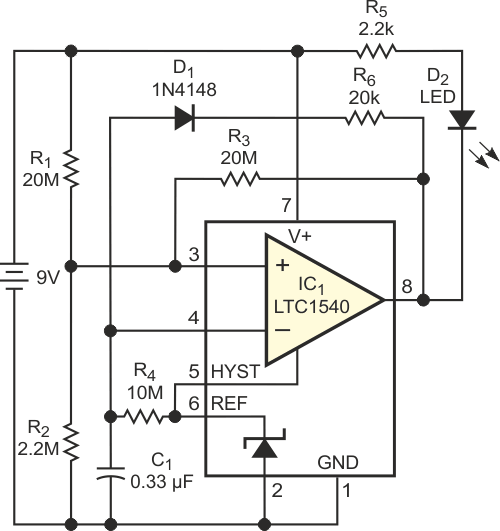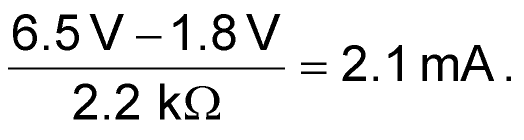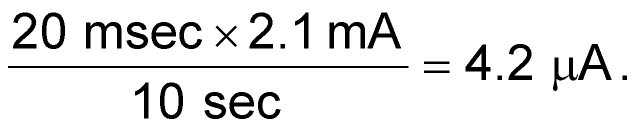# Low-battery indicator uses fleapower

## Analog Devices LTC1540

It is always desirable to use a low-battery indicator that consumes as little power as possible. For a 9 V, 450-mAhr alkaline battery, a 50-µA low-battery indicator can by itself run the battery down in a little more than a year. Battery-powered devices that need to run continuously for a long time require battery indicators that consume minimal power. The circuit in Figure 1, designed for a 9 V battery, uses extra-low power. When the battery is at full charge (9 V), the circuit draws 1.4-µA current. At the indication-threshold voltage, 6.5 V, the circuit draws 1 µA. Assume that the average operating current is 1.2 µA. The circuit uses 42 mAhr in a four-year period, less than 10% of the battery's rated energy. A red LED, D2, flashes periodically when the battery voltage drops below 6.5 V. IC1, an LTC1540, is a nanopower comparator with a built-in 1.18 V reference. A battery-voltage divider comprising R1 and R2, and a positive-feedback network, R3, feed the positive input of the comparator. The positive feedback generates hysteresis in the comparator. The negative input of the comparator receives bias from the reference voltage, through the R4 - C1 delay circuit.Figure 1. This fleapower low-battery indicator draws just 1.2-µA operating current.

During normal operation, the voltage at the positive input is approximately 1.62 V when the battery is at 9 V. The output of the comparator is at a high state, such that no current flows through D1 and D2. When the battery voltage drops below 6.5 V, the voltage at the positive input drops below the reference voltage at the negative input. The output of IC1 switches from high to low, thereby lighting the LED, D2. The switching changes the voltage at the positive input to 0.58 V and causes C1 to discharge through D1 and R6. Because the value of R6 is much smaller than that of R4, the voltage at the negative input drops quickly, according to the time constant that C1 and R6 set. Once the voltage at the negative input falls below 0.58 V, the comparator switches back to a high state. This change sets the voltage at the positive input to 1.18 V, turns off the LED, and reverse-biases D1, so the reference charges C1 through R4. When the voltage at the negative input again reaches 1.18 V, the cycle repeats. The LED's on- time is a function of C1 and R6, and the off-time is a function of C1 and R4. With the values in Figure 1, the on- and off-times are 20 msec and 10 sec, respectively, at 6.5 V threshold voltage.

At this point, the LED's on-state current isThe average LED current is## Materials on the topic

EDNYou may have to register before you can post comments and get full access to forum.
 User Name Remember Me? Password